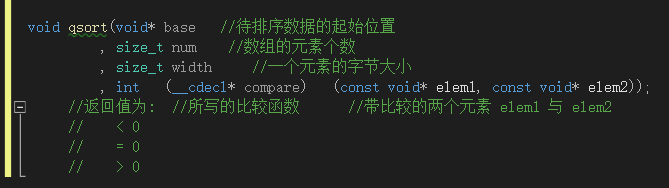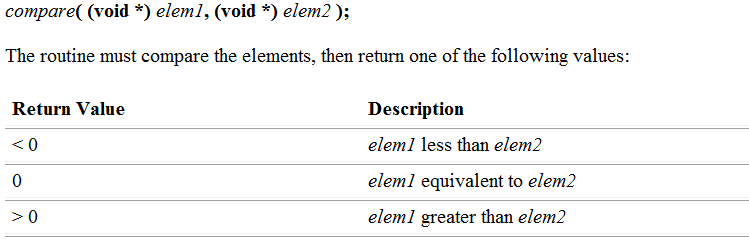## qsort函数（C语言）_qsort函数代码_川入的博客-程序员秘密

struct Stu（对比字符串）：

int：

char：

# 介绍：

qsort()函数是 C 库中实现的快速排序算法，包含在 `stdlib.h` 头文件中。函数原型如下：

``````void qsort( void *base, size_t num, size_t width, int (__cdecl *compare )(const void *elem1, const void *elem2 ) );

``````对于比较函数，因比较形式的多量化，所以作者就将比较函数交给书写者自行进行编译书写。（以整数类型数组排序举例）

``````#include <stdio.h>
#include <stdlib.h >

void print(int sz, int arr[])
{
int i = 0;
for (i = 0; i < sz; i++)
{
printf("%d ", arr[i]);
}
printf("\n");
}

int cmp_int(const void* e1, const void* e2)
{
return (int*)e1 - (int*)e2;
}

int main()
{
int arr = { 3,2,1 };
int sz = sizeof(arr) / sizeof(arr);
qsort(arr, sz, sizeof(arr), cmp_int);
print(sz, arr);
return 0;
}
``````

• cmp_int即为，为了进行整数数组的排序所进行的函数大小比较。
• qsort对我们所传入的数据判断，以我们所写的来进行断定。
``int (__cdecl *compare )(const void *elem1, const void *elem2 ) ``对此，我们知道qsort是一个运用，快排的方式，使得一串数据以低到高，如果，我们需要以高到低呢，这就需要反思维了，我们须知，以低到高，是有与前者大于后者即换，反之不换。那我们就反过来，后者大于前者即换，反之不换。那我们是不是就得到了一串有高到低的数据，于是我们将"e1 - e2";变为"e2 - e1"即可。以上者代码为例，我们将其变为如此即可：

``````#include <stdio.h>
#include <stdlib.h >

void print(int sz, int arr[])
{
int i = 0;
for (i = 0; i < sz; i++)
{
printf("%d ", arr[i]);
}
printf("\n");
}

int cmp_int(const void* e1, const void* e2)
{
return (int*)e2 - (int*)e1; //重点变化点
}  //-------------------------------

int main()
{
int arr = { 3,2,1 };
int sz = sizeof(arr) / sizeof(arr);
qsort(arr, sz, sizeof(arr), cmp_int);
print(sz, arr);
return 0;
}``````

# 不同类型

## struct Stu（对比字符串）：

``````#include <stdio.h>
#include <stdlib.h>

struct Stu
{
char name;
int age;
};

int cmp_struct_Stu(const void* e1, const void* e2)
{
return strcmp(((struct Stu*)e1)->name, ((struct Stu*)e2)->name);
}

int main()
{
struct Stu arr = { {"zhangsan", 20},{"lisi", 30},{"wangwu", 10} };
int sz = sizeof(arr) / sizeof(arr);
qsort(arr, sz, sizeof(arr), cmp_int);
int i = 0;
for (i = 0; i < sz; i++)
{
printf("%s,%d   ", (arr[i]).name, (arr[i]).age);
}
printf("\n");
return 0;
}``````

## int：

``````#include <stdio.h>
#include <stdlib.h>

void print(int sz, int arr[])
{
int i = 0;
for (i = 0; i < sz; i++)
{
printf("%d ", arr[i]);
}
printf("\n");
}

int cmp_int(const void* e1, const void* e2)
{
return (int*)e1 - (int*)e2;
}

int main()
{
int arr = { 3,2,1 };
int sz = sizeof(arr) / sizeof(arr);
qsort(arr, sz, sizeof(arr), cmp_int);
print(sz, arr);
return 0;
}``````

## char：

``````#include <stdio.h>
#include <stdlib.h >

int cmp_char(const void* e1, const void* e2)
{
int n = (*(char*)e1 - *(char*)e2);
return (*(char*)e1 - *(char*)e2);
}

void print(int sz, char arr[])
{
int i = 0;
for (i = 0; i < sz; i++)
{
printf("%c ", arr[i]);
}
printf("\n");
}

int main()
{
char arr = { 'b','a','c'};
int sz = sizeof(arr) / sizeof(arr);
qsort(arr, sz, sizeof(arr), cmp_int);
print(sz, arr);
return 0;
}``````

import的几种用法

### python模块paramiko与ssh安装配置教程_paramico配置sh_程序员如山石的博客-程序员秘密

paramiko是用python语言写的一个模块,遵循SSH2协议,支持以加密和认证的方式,进行远程服务器的连接了，下面我们就来给各位一起验证一下python模块paramiko与ssh安装与使用示例吧。一、paramiko模块的安装paramiko模块依赖PyCrypto模块，而PyCrypto需要GCC库编译，不过一般发行版的源里带有该模块。这里以centos6为例，直接借助以下命

### 聚类一之距离聚类_zhao_crystal的博客-程序员秘密

1. 聚类的定义聚类就是对大量未知标注的数据集，按数据的内在相似性将数据集划分为多个类别，使类别内的数据相似度较大而类别间的数据相似度较小。2. 聚类的应用2.1 降维对于M篇文章，D1， D2， D3 …… Dm，假设已做好分词。根据这M篇文章，得到一个词典word_dict = {W1, W2, W3, W4……Wv},假设共有v个词。方式1: 0，1 矩阵（0代表没有出现过，1代表出现过）若D1中出现过W1，则将相应位置置为1，否则置为0。其它同理。——&gt;m纬..